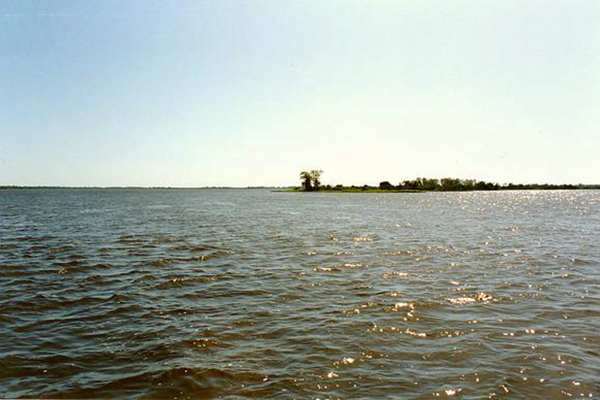Upper Paraguay river at Porto Murtinho, Mato Grosso do Sul, Brazil, featuring a flood hydrograph lasting one year (the maximum possible), clearly the quintessential flood wave.

WHEN IS THE DIFFUSION WAVE APPLICABLE?

Victor M. Ponce

Professor Emeritus of Civil and Environmental Engineering

San Diego State University, San Diego, California

25 August 2023

 ABSTRACT.  A review of diffusion waves and their use for routing flood waves has been accomplished. Diffusion waves travel with the Seddon celerity, i.e., the kinematic wave celerity, and are subject to little attenuation (diffusion). These properties distinctly match those of typical flood waves. Other free-surface flow waves, namely, kinematic, mixed, and dynamic, are either nondiffusive (kinematic and dynamic), or too diffusive (mixed). In particular, the mixed waves are confirmed to be so greatly diffusive as to question their mere existence altogether. Criteria for the applicability of both kinematic and diffusion waves show that the latter, the diffusion waves, have a broader range of applicability than do the kinematic waves. Therefore, the diffusion wave is recommended for practical applications in flood hydrology.

1.  INTRODUCTION

The diffusion wave is a type of wave used in flood routing. Other types of waves are the kinematic wave and the mixed kinematic-diffusion wave, hereafter called "mixed wave" (Lighthill and Whitham, 1955; Ponce and Simons, 1977). Note that the mixed wave has been widely referred to in the literature as "dynamic wave", although this usage appears to be ill-advised, because it leads to a semantic confusion with the long-established dynamic wave of Lagrange (1788), a concept quite different from the mixed wave.

A comprehensive classification of shallow-water waves in open-channel flow was accomplished by Ponce and Simons (1977), who used linear stability theory to derive the celerity and attenuation functions of all four types of shallow-water waves: (1) kinematic waves, (2) diffusion waves, (3) mixed waves, and (4) dynamic waves. These wave types are defined in terms of the dimensionless wavenumber σ*, as shown in Fig. 1 (Ponce, 2023). Kinematic waves correspond to the smallest values of σ* (to the left of the scale), and dynamic waves to the largest (to the right of the scale). Mixed waves lie along the middle-to-right of the scale, while diffusion waves lie along the left-of-middle.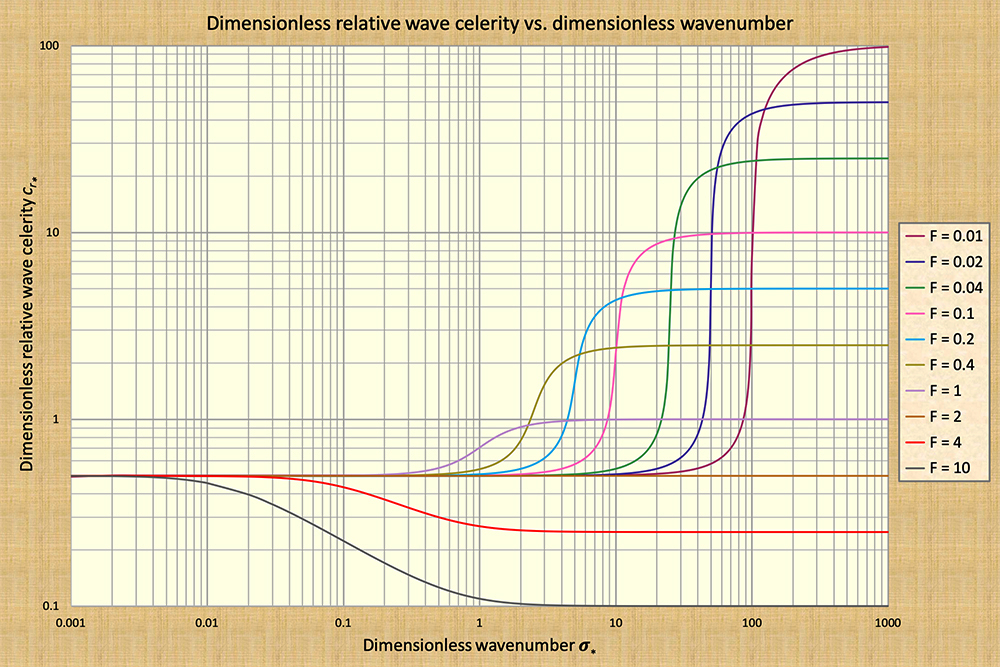Ponce and Simons (1977)

Fig. 1   Dimensionless relative wave celerity cr* vs dimensionless wavenumber σ*.

In Figure 1, kinematic waves lie along the first log cycle (to the left), while dynamic waves lie along the fifth and sixth cycles (to the right), depending on the Froude number. Mixed waves lie mostly along the fourth and fifth cycles, while diffusion waves lie along the second and third cycles.

Hydrodynamic theory asserts that if the wave celerity is a constant along the dimensionless wavenumber, wave attenuation is zero. In Figure 1, this condition corresponds to both kinematic waves (left of scale) and dynamic waves (right of scale). Conversely, if the wave celerity varies along the dimensionless wavenumber, as in the case of a mixed wave, wave attenuation is nonzero. Wave attenuation reaches a maximum at the point of zero curvature of the dimensionless relative celerity function cr* vs dimensionless wavenumber σ* (Fig. 1), i.e., when the second derivative is equal to zero. Experience indicates that these maximum values of wave attenuation may actually render the wave in question nonexistent, due to the extremely high rates of attenuation (Lighthill and Whitham, 1955).

Thus, the question remains: If the kinematic waves have no attenuation, and the mixed waves are subject to very strong attenuation, what happens to the diffusion waves, which ostensibly lie in between them? The answer is: The diffusion waves are subject to a small but finite amount of attenuation, which is generally much smaller than the strong attenuation featured by mixed waves.

In this article, we make the case for the diffusion wave. We note that if the flood wave has a small amount of attenuation, the diffusion wave model will account for it, while the kinematic wave model will not. Furthermore, we show that due to the large amounts of attenuation which are predicted for mixed waves, the latter are not very likely to occur in the real world. In Section 2, we explain the nature of flood waves and make a point of the need to focus on the diffusion wave. With the applicability question clearly answered in Section 4, the time has come to hail the diffusion wave as the method of choice in flood routing engineering practice.

2.  NATURE OF A FLOOD WAVE

What is the nature of a flood wave? Essentially, a flood wave is a "long" wave, i.e., one of small dimensionless wavenumber (Fig. 1), traveling at, or very close to, the kinematic wave celerity, and experiencing little attenuation. Seddon (1900) pioneered the study of flood waves, concluding that its celerity could be expressed as the slope of the discharge-area rating Q = αAβ, in which Q = discharge, A = flow area, and α and β are coefficient and exponent, respectively.

According to Seddon, the celerity of a flood wave is: c = dQ/dA, in which dA = (1/T)dy, with T = (stream) channel top width, and y = stage, or water surface elevation. He expressed the celerity of a flood wave as c = (1/T) dQ/dy. Thus, a flood wave is essentially a kinematic wave subject to a relatively small amount of diffusion. Indeed, this is the kinematic-wave-with-diffusion of Lighthill and Whitham (1955) or, more concisely, the diffusion wave of Ponce and Simons (1977).

As long as diffusion needs to be accounted for, diffusion waves may not be modeled with kinematic waves, because the latter feature zero diffusion. We note that in the 1980s, kinematic waves were solved using numerical models, and the latter did feature some diffusion. This diffusion, however, was uncontrolled numerical diffusion, and not related to the true physical diffusion of the flood wave; therefore, the results of the routing varied with the choice of grid size.

Could the flood wave be construed as a mixed wave, a wave that sits on the middle-to-right of the dimensionless wavenumber spectrum (Fig. 1)? The answer is: Not likely for typical flood waves, which hold their stage and do not attenuate very much. If the flood wave were to attenuate strongly, it would cease to be a flood wave, instead joining the mass of the underlying equilibrium, steady flow. Thus, we conclude that mixed waves are not an appropriate model of flood waves, at least, not in the general case. In the following section, calculations of diffusion waves will confirm these statements.

Having placed aside: (a) the kinematic waves, because they lack diffusion entirely, and (b) the mixed waves, because they have too much diffusion, we are left only with the diffusion wave, which lies in between kinematic and mixed waves in the dimensionless wavenumber spectrum. This is the wave that truly embodies the nature of flood waves: A kinematic wave featuring a small, but perceptible, amount of diffusion.

3.  THE DIFFUSION WAVE

According to theory, the dimensionless relative celerity of a diffusion wave resembles that of a kinematic wave, but unlike the latter, it increases, ever so slightly with the dimensionless wavenumber σ* (Fig. 1). This increase is the source of the wave diffusion which characterizes the diffusion wave.Ponce and Simons (1977)

Fig. 1   Dimensionless relative wave celerity cr* vs dimensionless wavenumber σ*.

The amount of wave diffusion is expressed in terms of the logarithmic decrement δ, a measure of the rate at which the wave changes upon propagation (Wylie, 1966). The definition of logarithmic decrement is: δ = ln Q1 - ln Q0, or, alternatively: Q1 = Q0 eδ, in which Q0 = flood discharge at the start of the measurement, and Q1 = flood discharge after an elapsed time equal to one (sinusoidal) period of propagation.

The discharge decreases for a negative value of δ, causing wave attenuation, corresponding to Froude number F < 2 (Vedernikov number V < 1); it increases for a positive value, a logarithmic increment, causing wave amplification, corresponding to Froude number F > 2 (V > 1) (Ponce, 1991).

Ponce and Simons (1977) have used linear stability theory to calculate the logarithmic decrement of the diffusion wave. The expression is: δd = (2 π /3) σ*. Note that in this expression, as σ* → 0, the logarithmic decrement δd → 0, confirming that a kinematic wave is not subject to attenuation. Moreover, note that for σ* → ∞, the diffusion wave logarithmic decrement δd → ∞, confirming the inability of the diffusion wave logarithmic decrement formula to account for the dynamic waves, which also feature zero attenuation.

Figure 2 shows the variation of the logarithmic decrement for all wave types, through the range of dimensionless wavenumbers from 0.001 to 1000, for Froude numbers F < 2. Moreover, Figure 3 shows the variation of the logarithmic increment for all wave types, through the range of dimensionless wavenumbers from 0.001 to 1000, for Froude numbers F > 2.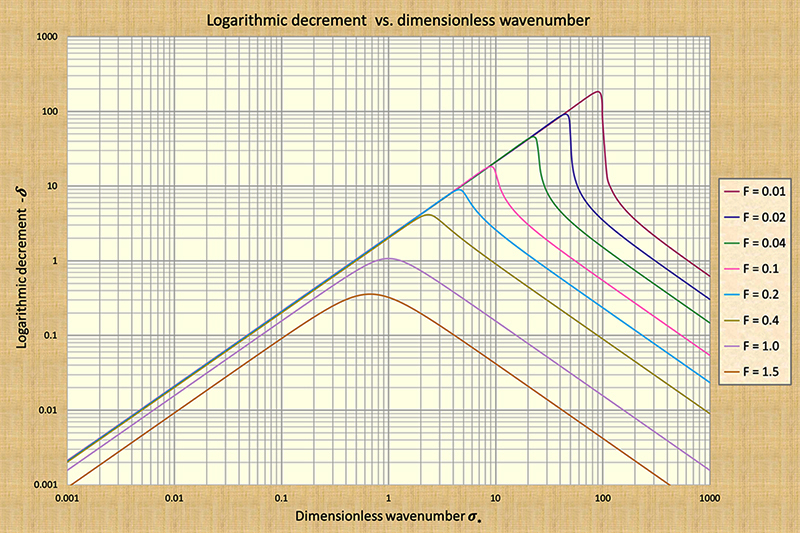Ponce and Simons (1977)

Fig. 2   Logarithmic decrement -δ  vs dimensionless wavenumber σ* for Froude F < 2.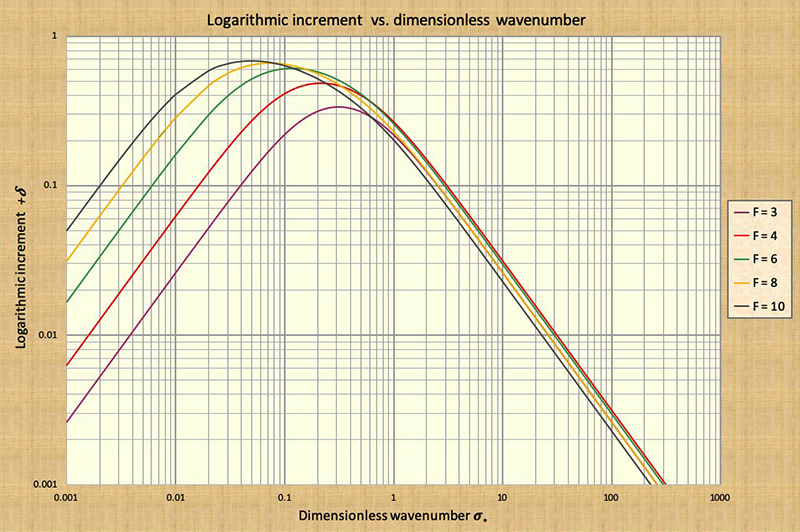Ponce and Simons (1977)

Fig. 3   Logarithmic increment +δ  vs dimensionless wavenumber σ* for Froude F > 2.

Table 1 shows values of the diffusion wave logarithmic decrement δd  relevant in the present context. The examination of this table leads to the conclusions summarized in Box A.

 Table 1. Logarithmic decrement of the diffusion wave across the dimensionless wavenumber spectrum.       No. Dimensionless wavenumber σ* Logarithmic decrement δd eδd Wave attenuation (1 - eδd) Wave type 0 0.0001 0.00020944 1 0 Kinematic 1 0.001 0.0020944 0.998 0.002 Kinematic/diffusion 2 0.01 0.020944 0.979 0.021 Diffusion 3 0.1 0.20944 0.811 0.189 Diffusion 3a 0.17 0.35604 0.700 0.300 Diffusion/Mixed 4 1 2.0944 0.123 0.877 Mixed 5 10. 20.944 0 1 Mixed 6 100. N.A. N.A. N.A. Dynamic 1 7 1000. N.A. N.A. N.A. Dynamic 1 1 In Col. 6, lines 6 and 7 are labeled as "Dynamic". The diffusion wave logarithmic decrement δd does not apply after the peak of attenuation is reached, i.e., in the dynamic wave range (see Fig. 2).

 Box A.  Conclusions from Table 1. For very small dimensionless wavenumbers (see Line 0), the wave attenuation, shown in Col. 5, is zero, i.e., a kinematic wave. For small dimensionless wavenumbers (see Line 2), the wave attenuation, shown in Col. 5, is 0.021 (2.1%) , i.e., a diffusion wave. Wave attenuation reaches 0.3, a high number (30%) for σ* = 0.17; i.e., at the limit between diffusion and mixed waves. Wave attenuation reaches 0.877, a very high number (87.7%), for σ* = 1 , i.e., a mixed wave. Wave attenuation reaches 1, the maximum value (100%), for σ* = 10, i.e., a mixed wave.

We confirm that kinematic waves are not subject to attenuation. We also confirm that for the midrange value of dimensionless wavenumber σ* > 0.17, the wave attenuation is greater than 30%, a threshold which is widely considered to be the division between diffusion waves (of limited wave diffusion, less than 30%) and mixed waves (of unlimited wave diffusion, which could readily reach 100%) (Flood Studies Report, 1975). Thus, the rationale for the argument that mixed waves are very strongly dissipative and, in most cases of practical interest, that they will not likely be there for us to calculate them (Ponce, 1992).

We confirm the following observations, based on detailed calculations, in reference to Fig. 1: (a) kinematic waves lie along the first log cycle; (b) diffusion waves along the second and third, (c) mixed waves along the fourth and fifth, and (d) dynamic waves along the fifth and sixth cycles, the latter depending heavily on the Froude number. Kinematic waves do not attenuate, mixed waves attenuate very strongly, and dynamic waves, being characteristically short, do not resemble flood waves, which are demonstrably long. Thus, a strong case is made for the applicability of the diffusion wave for flood routing applications.

4.  APPLICABILITY OF DIFFUSION WAVES

Having shown that diffusion waves are applicable to the flood routing problem, we now show just how applicable they are. Here we elaborate on the work of Ponce and others (1978), who established the criterion for the applicability of kinematic and diffusion waves in terms of flood wave properties.

For the kinematic wave model, Ponce and others stated that to achieve at least 95% accuracy of the kinematic wave solution after one period of propagation, the dimensionless period has to satisfy the following inequality: τ* ≥ 171. The dimensionless period is defined as follows: τ* = T So (uo /do), in which T = period of the perturbation, So = channel (stream) bottom slope, uo = mean equilibrium flow velocity, and do = equilibrium flow depth. For example, given the following data: uo = 3 fps, do = 10 ft, and So = 0.0001, the resulting wave period is: T > (171 × 10) / ( (0.0001 × 3) = 5,700,000 seconds = 65.97 days. In other words, the duration of the flood must be greater than about 66 days for the kinematic wave solution to be at least 95% accurate after one period or propagation. It follows that the longer the flood duration, the more kinematic the flood wave is, and this finding agrees admirably with the theory.

For the diffusion wave model, Ponce and others compared the logarithmic decrement of the diffusion wave δd with that of the full solution (Fig. 2), and concluded that to achieve at least 95% accuracy of the diffusion wave solution after one period of propagation, the dimensionless period has to satisfy the following inequality: τ* ≥ 30. In this case, the dimensionless period is defined as follows: τ* = T So (g /do)1/2, in which g = gravitational acceleration. Using the previous example for comparison, for do = 10 ft, and So = 0.0001, the resulting wave period is: T > 30 / ( (0.0001 × [32.17/10)1/2] = 167,261 seconds = 1.93 days. In other words, the duration of the flood wave must be greater than 1.93 days (about 2 days) for the diffusion wave solution to be at least 95% accurate after one period or propagation. This finding reveals that the theory of diffusion waves is correct: These waves will apply for a larger number of cases than the kinematic wave. For the example presented here, a flood wave would need to have a minimum duration of 66 days for the kinematic wave to apply; however, for the diffusion wave, a minimum of only 2 days will suffice. Thus, we confirm the broad range of practical cases for which the diffusion wave is applicable.

5.  APPLICATION TO FIELD DATA

The concepts elaborated in the previous section are applied here to the Upper Paraguay river, in Mato Grosso do Sul, Brazil, and neighboring Bolivia. This river features a unique geographical setting, flowing through a continental delta encompassing the Pantanal of Mato Grosso, the largest wetland in the world, spanning 136,700 km2. The geomorphological and hydrological setting of the Upper Paraguay river has been described by Ponce (1995).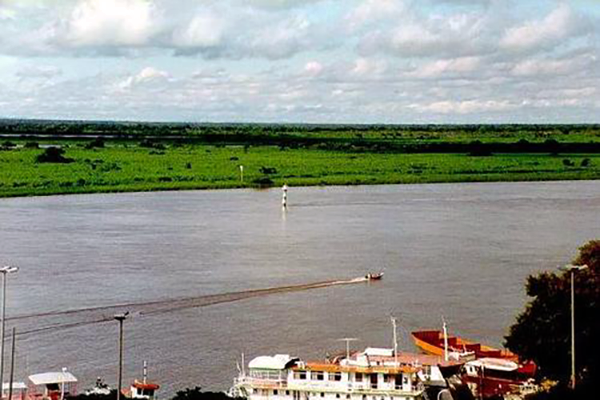Fig. 4  Upper Paraguay river near Ladario, Mato Grosso do Sul, Brazil.

The flood hydrograph of the Upper Paraguay river, in its lower reaches, from Ladario to Porto Murtinho, comprising a distance of 520 km along the river, features only one peak flow stage, a condition attributed to the extreme runoff diffusion caused by the very small slope of the river channel, which varies between 2.34 cm/km near Ladario, and 0.83 cm/km near Porto Murtinho, for an average of 1.6 cm/km.

Downstream of Ladario, the river stage rises from March to August, receding from September to February. The peak stage occurs typically in the month of June and the low stages in December. The mean flow depth varies between 5.24 m at Ladario and 12.28 m at Porto Murtinho, for an average of 8.76 m. Moreover, the mean speed of propagation of the flood wave has been estimated at 0.1 m/s (Ponce, 1995). Thus, the values relevant to the assessment of flood wave model applicability are the following: T = 12 months, So = 0.000016, uo = 0.1 m/s, and do = 8.76 m. The calculations are summarized in Box B.Fig. 5  Upper Paraguay river near Porto Murtinho, Mato Grosso do Sul, Brazil.

 Box B.  Summary of calculations of flood wave model applicability. Relevant data: T = 12 months, So = 0.000016, uo = 0.1 m/s, and do = 8.76 m. Kinematic wave applicability relation: τ* = T So (uo /do) ≥ 171 τ* = 12 mo × 30 days/mo × 86,400 sec/day × 0.000016 × 0.1 m/sec / 8.76 m = 5.68 τ* = 5.68 << 171. Therefore, the kinematic wave model is not applicable. This is attributed to the very mild channel slope (1.6 cm/km). Diffusion wave applicability relation: τ* = T So (g /do)1/2 ≥ 30. τ* = 12 mo × 30 days/mo × 86,400 sec/day × 0.000016 × (9.81 m/sec2 / 8.76 m)1/2 = 527 τ* = 527 >> 30. Therefore, the diffusion wave model is applicable. Even with this very small channel slope (1.6 cm/km), the diffusion wave model remains applicable.

6.  CONCLUDING REMARKS

A review of diffusion waves and their use for routing flood waves has been accomplished. Diffusion waves travel with the Seddon celerity, i.e., the kinematic wave celerity, and are subject to little attenuation (diffusion). These properties distinctly match those of typical flood waves. Other free-surface flow waves, namely, kinematic, mixed, and dynamic, are either nondiffusive (kinematic and dynamic), or too diffusive (mixed). In particular, the mixed waves are confirmed to be so greatly diffusive as to question their mere existence altogether. Criteria for the applicability of both kinematic and diffusion waves show that the latter, the diffusion waves, have a broader range of applicability than do the kinematic waves. Therefore, the diffusion wave is recommended for practical applications in flood hydrology. An application to field data from the Upper Paraguay river, in Mato Grosso do Sul, Brazil, further confirms the findings of this study.

REFERENCES

Lagrange, J. L. de. 1788. Mécanique Analytique, Paris, part 2, section II, article 2, 192.

Seddon, J. A. 1900. River hydraulics. Transactions, ASCE, Vol.XLIII, 179-243, June.

Lighthill, M. J. and G. B. Whitham. 1955. On kinematic waves. I. Flood movement in long rivers. Proceedings, Royal Society of London, Series A, 229, 281-316.

Wylie, C. R. 1966. Advanced Engineering Mathematics, 3rd ed., McGraw-Hill Book Co., New York, NY.

Flood Studies Report. 1975. Vol. III: Flood Routing Studies, Natural Environment Research Council, London, England.

Ponce, V. M. and D. B. Simons. 1977. Shallow wave propagation in open channel flow. Journal of the Hydraulics Division, ASCE, 103(12), 1461-1476.

Ponce, V. M., R. M. Li, and D. B. Simons. 1978. Applicability of kinematic and diffusion models. Journal of the Hydraulics Division, ASCE, 104(3), 353-360.

Ponce, V. M. 1991. New perspective on the Vedernikov number. Water Resources Research, Vol. 27, No. 7, 1777-1779, July.

Ponce, V. M. 1992. Kinematic wave modeling: Where do we go from here? International Symposium on Hydrology of Mountainous Areas, Shimla, India, May 28-30.

Ponce, V. M. 1995. Hydrologic and environmental impact of the Parana-Paraguay waterway on the Pantanal of Mato Grosso, Brazil. https://ponce.sdsu.edu/hydrologic_and_environmental_impact_of_the_parana_paraguay_waterway.html

Ponce, V. M. 2023. Kinematic and dynamic waves: The definitive statement. Online article.

 230825 13:00 PDT